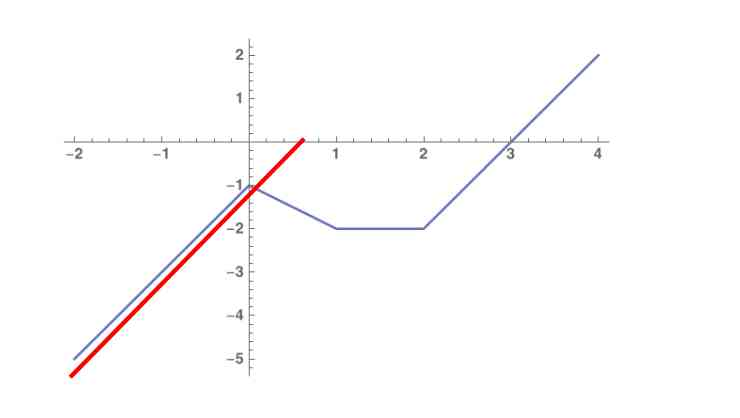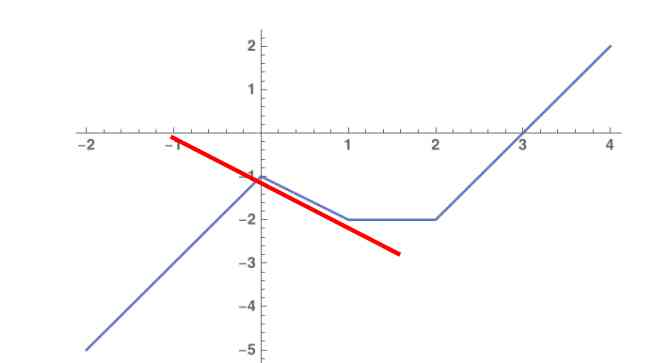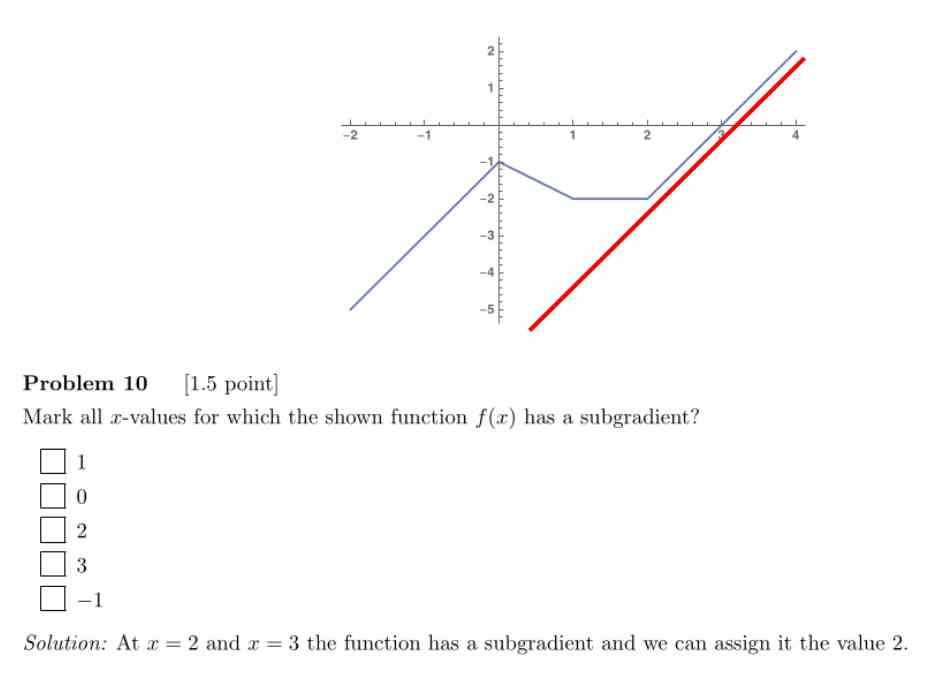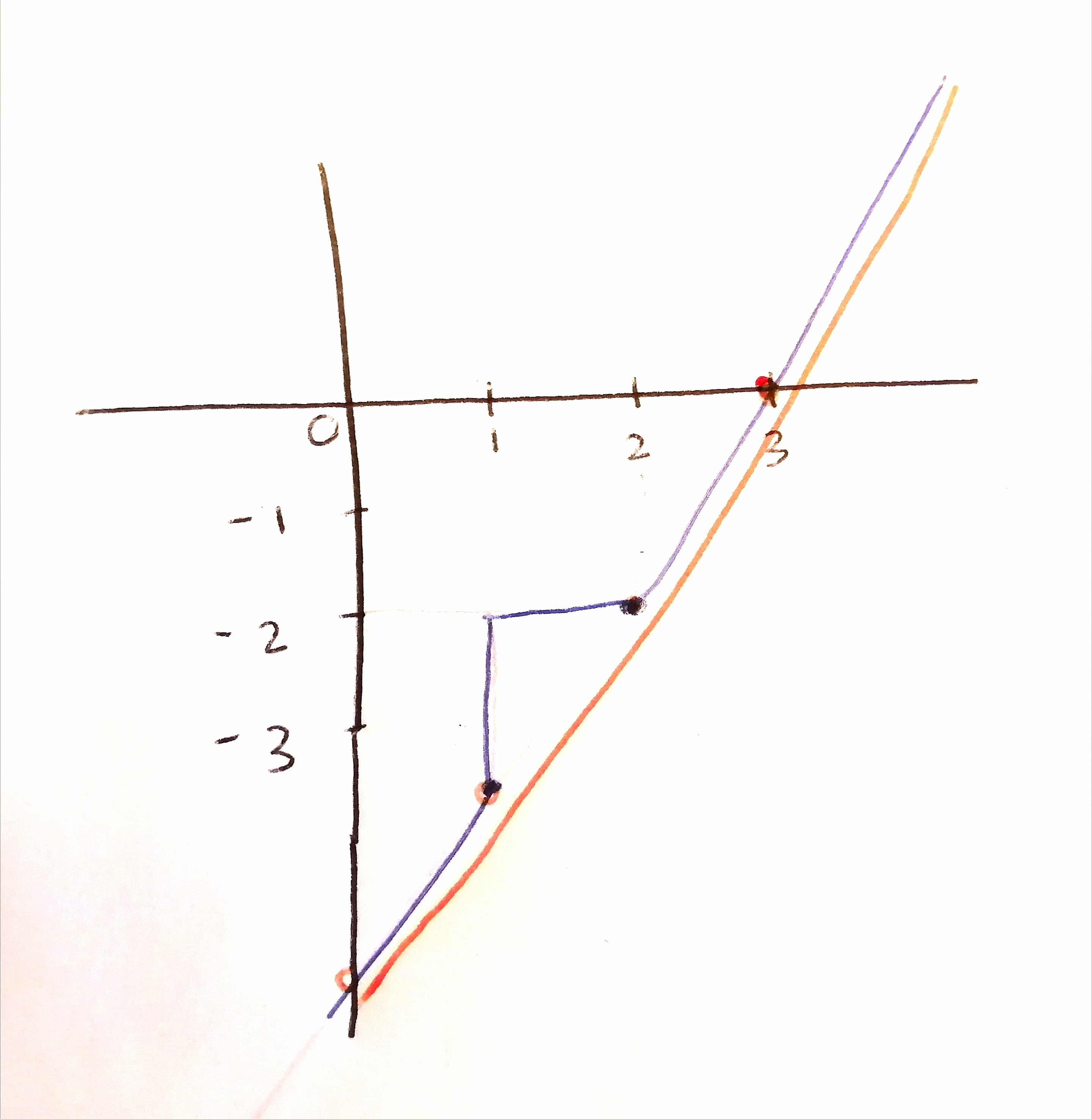According to the definition of subgradient

$$\mathcal{L}(\mathbf{u}) \geq \mathcal{L}(\mathbf{w})+\mathbf{g}^{\top}(\mathbf{u}-\mathbf{w}) \quad \forall \mathbf{u}$$

Could we define the following gradients?
A. From [-2,0]
It is touching the function and it is bellow the functionB. From [0,1]
It is touching the function and it is bellow the functionThank you in advance :)

these look reasonable only in a local neighborhood, but they do not satisfy the actual subgradient definition you stated (the 'for all u' part is meant global)

Thank you very much for answering :)

In this case, only x = 2 and x = 3 have a subgradient because it is the only subgradient that is globally bellow the function for [-2,4]?In the following function, can we say that the function has a subgradient with value 2 at x = 0, x = 1, x = 2 and x = 3?Page 1 of 1